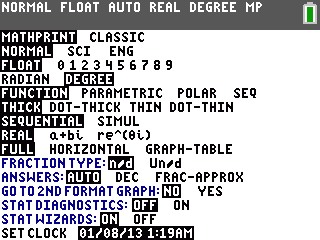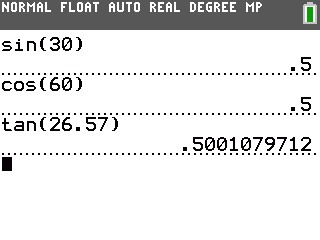# Knowledge Base

## Solution 34771: Calculating Sine, Cosine, and Tangent on the TI-83 Plus and TI-84 Plus Family of Graphing Calculators.

### How do I calculate sine, cosine, and tangent on the TI-83 Plus and TI-84 Plus family of graphing calculators?

The following examples will demonstrate how to compute sine, cosine, and tangent.

Please Note: For these examples, the calculator's angle setting will need to be changed.

1) Press [MODE] key.
2) Scroll down to "RADIAN", scroll right to highlight "DEGREE" and press [ENTER]. This will change the calculator's angle setting to Degree mode.For Example: Calculate the sine of 30°.

1) Press [SIN]  .
2) Press [)] [ENTER].

For Example: Calculate the cosine of 60°.

1) Press [COS]  .
2) Press [)] [ENTER].

For Example: Calculate the tangent of 26.57°.

1) Press [TAN]   [.]  .
2) Press [)] [ENTER].Please Note: Since the calculator's angle setting is set to Degree, there's no need to input the degree symbol ( ° ) behind the value.

Please see the TI-83 Plus and TI-84 Plus Family guidebooks for additional information.

Last updated: 6/29/2023